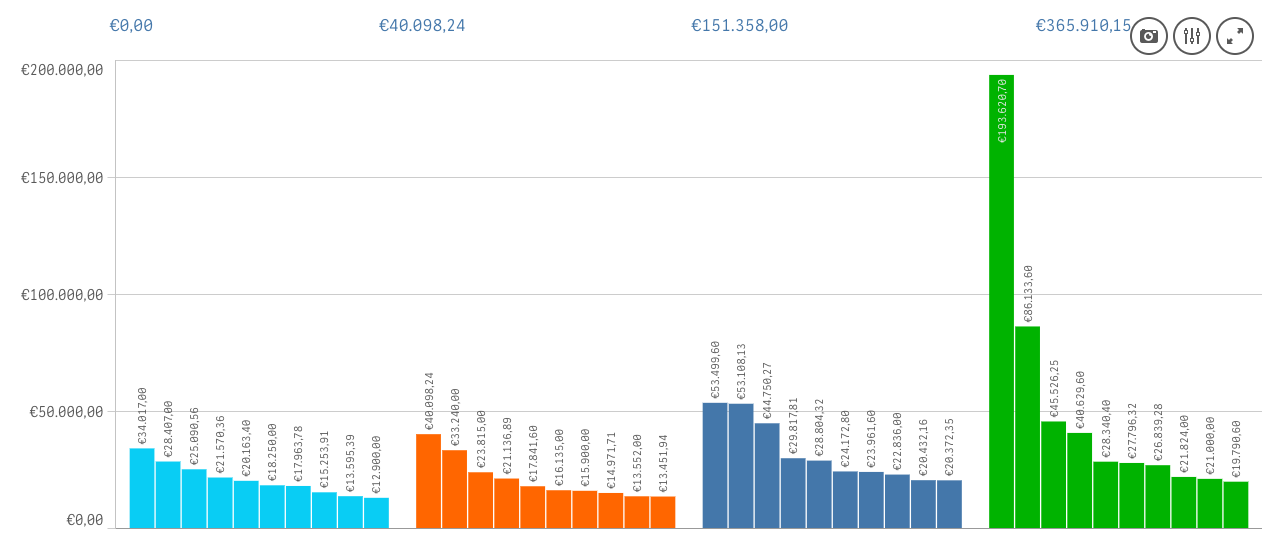# New to Qlik Sense

Discussion board where members can get started with Qlik Sense.

Announcements
Talk to Experts, a LIVE Q&A Webinar. Bring your Qlik Sense Business questions on Aug. 4th. Register
cancel
Showing results for
Did you mean:
HighlightedCreator II

## Rank function with multiple dimensions

Hi experts,

I've made a bar chart with my top 10 invoices over the last 4 years.

Because Qlik doesn't have a function that shows the total sum of these top 10 customers per year, I wanted to use a KPI object to show the total sum of the top 10 invoices in a certain year.

See the following screenshot (Years are ordered as 2014-2017).In the 4 different KPI objects I used the following function 4 times (Max Year, Max Year -1, -2, -3):

 sum({1}Year))"},[Invoice Number] = {"=rank(sum([Nett Amount new EUR]),4,1) <= 10"}>} [Nett Amount new EUR] )

The numbers shown in the KPI objects are based on the top 10 invoices over the 4 years, instead of the top 10 invoices per year.

Could you guys help me out with this one or give me another solution?

Mike

1 Solution

Accepted Solutions
HighlightedMVP

I removed the double quotes within the Max({<year>}Year) thing... can you check... also do you have two year fields? Year and year? QlikView is case sensitive, make sure to use the correct casing everywhere

Sum({1<Year={"\$(=Max({<Year>} Year))"}, [Invoice Number] = {"=Rank(Sum({1<Year={\$(=Max({<Year>} Year))}>} [Nett Amount new EUR]), 4, 1) <= 10"}>} [Nett Amount new EUR])

6 Replies
HighlightedMVP

May be this

Sum({1<Year={"\$(=Max({<year>} Year))"}, [Invoice Number] = {"=Rank(Sum({1<Year={\$(=Max({<year>} Year))}>} [Nett Amount new EUR]), 4, 1) <= 10"}>} [Nett Amount new EUR])

HighlightedCreator II

Thanks for your quick reply Sunny, but this function is showing a NULL value " - "..

HighlightedMVP

I removed the double quotes within the Max({<year>}Year) thing... can you check... also do you have two year fields? Year and year? QlikView is case sensitive, make sure to use the correct casing everywhere

Sum({1<Year={"\$(=Max({<Year>} Year))"}, [Invoice Number] = {"=Rank(Sum({1<Year={\$(=Max({<Year>} Year))}>} [Nett Amount new EUR]), 4, 1) <= 10"}>} [Nett Amount new EUR])

HighlightedCreator II

This did the job! Thanks a lot Sunny, you helped me a lot!:)

HighlightedPartner

Hi Sunny,

Can you please explain to me what does the 4 and 1 do?

Rank(Sum({1<Year={\$(=Max({<Year>} Year))}>} [Nett Amount new EUR]), 4, 1) <= 10

Thanks !

HighlightedMVP